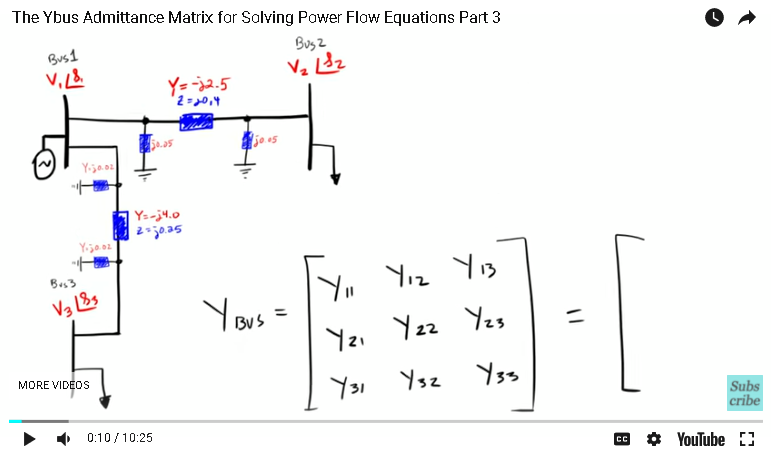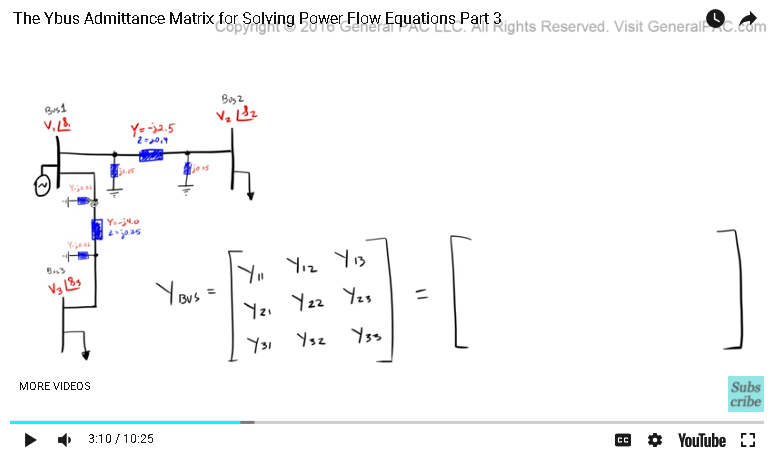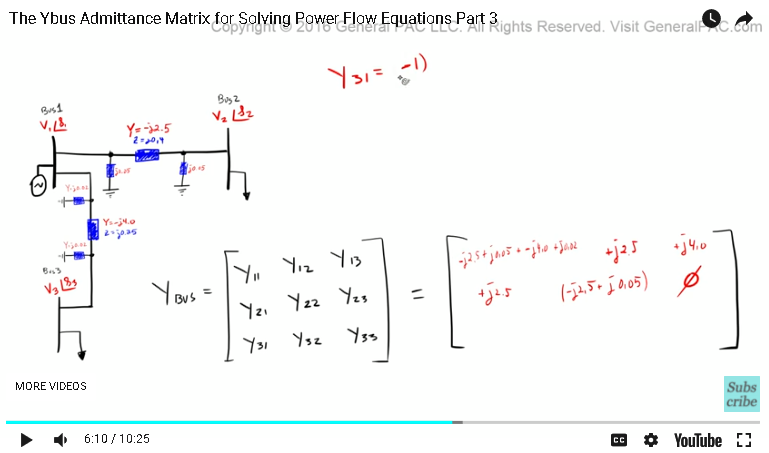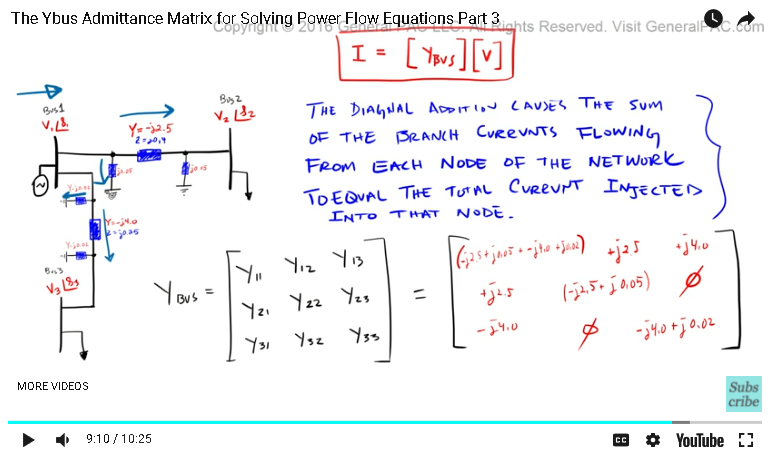This video was brought to you by GeneralPAC.com, making power systems Intuitive, Open and Free for Everyone, Everywhere. Consider subscribing and supporting through patreon.com/GeneralPAC. This is a mechanism for you to support us financially so we can continue making high quality power system video tutorials. Our corporate sponsor for this topic is AllumiaX.com from Seattle, Washington. Contact them for industrial and commercial power system studies.

The Tutorial on the YBus Admittance Matrix for Power Flow Equations Part Three.

So, in part two, we went over a very generalized example of YBus admittance matrix for the power system, and we drew some conclusions from it, right?One conclusion that we drew was that the diagonal element, which are Y11, Y22 and Y33. These diagonal elements represent the admittance of a particular Bus, right? And we'll talk about what that means in a little while. But it essentially equals the sum of all of the admittances connected to that bus, right?

So, Y11, Y22 and Y33, so the sum of admittances that's connected to bus one, the sum of admittances that are connected to bus two, the sum of admittances that are connected to bus three. Whereas, the off diagonal elements, which are these guys right here, Y12, Y13, Y21, Y23 and Y31, Y32, these are the negative, so we've had to have a negative terms here. Multiply that by the admittances that spans from that bus to the second bus.

So, let's consider Y12. So, Y12 is going to equal negative one times the admittance from bus one to bus two. Well, here's bus one and here's bus two. So, we're talking about the admittance that spans from bus one to bus two, which is this admittance here, right? And that is equal to negative J two point five.

So, Y12 is going to equal negative one times negative J two point five and that's going to equal positive J two point five, okay?

Similarly, we have to look at the admittance that spans bus one and three. In this example, that admittance is this guy right there. Y13 is going to equal negative one times that admittance, which is J four point zero and that is going to equal positive J four point zero, okay? So, these are the off diagonal elements.

So, let's keep this video short and let's just fill out our matrix, okay? So, now we're going to start by calculating the admittance matrix for bus Y11. So, we look at bus one and then we look at all of the admittances that are connected to bus one. So, bus one, there are a total of four admittances, right? We have the admittance of this transmission line, which is negative two point five. We have the shunt admittance of this transmission line, which is point zero five. The admittance of this transmission, which is negative four point 0, and the shunt admittance of this transmission line, which is J point zero two.So Y11 is going to equal J two point five, and that's a negative value, right? Plus J zero point zero five, which is this admittance here, plus negative J four point zero, which is this value here and then plus this guy right there, J zero point zero two. Okay?

So, we add all of this, all of these sums up and then we put that over here, okay? That's Y11. Pretty straight forward, right?

So, now Y12, that's going to equal the admittance negative one, right? Times the admittance from bus one to bus two, which is negative two point five, so negative J two point five, and that's going to equal positive J two point five, okay?

Make this smaller, that's going to be there, okay? And then Y13, that's going to equal, because it's an off diagonal element, we're going to multiply by negative one, that's the admittance from bus one to bus three, which is negative J four point zero, positive J four point zero. Put that right there, okay.

Now, similarly, Y21 is the admittance from bus two to bus one, and I have that negative one there, so that is equal to negative two point five, positive, put that there, and then Y22, that's going to equal all of the admittances that are connected to bus two and the only two admittances that are connected to bus two are this line admittance plus this shunt admittance. So, that's going to equal negative J two point five, that's the line admittance, plus the shunt admittance, which is J zero point zero five. So, that's going to equal that.

And then Y23, that's going to equal the admittance from bus two to bus three. Now notice, this is bus two here and this is bus three there, there's no admittance that's connected between bus two and bus three, so that value is just going to equal zero. So, we're going to have zero there, okay?

And admittance for Y31, that's going to equal the admittance from bus three to bus one, which is negative J ... Remember it's an off diagonal value, so that's going to equal negative J four point zero, put it there. And then Y32, which is the admittance from bus three to bus two, remember, nothing there. No admittances that are connected there, so that's going to equal zero and then Y33 are the admittances that are connected to bus three, which is only these two admittances. So, we have negative J four point zero, plus J zero point zero two. Put that there.So, there you have it. For this particular example, the YBus matrix is equal to that right there.

Now remember in part two, we said that the current is equal to the YBus matrix. Times that by our voltage, remember in the previous tutorial, this is essentially what we concluded if we modeled out the currents. So now, the question that I posed in part two was, in the diagonal matrices, why is it that we're actually adding up all of the admittances that are connected to that particular bus? And the answer to that question is this.

Okay, so we're saying that the diagonal addition causes the sum of the branch currents flowing from each node of the network to equal the total current injected into that node. Now, this definition comes directly from the Grainger and Stevenson's Power Systems Analysis book, and at first it was a pretty difficult thing to understand, but it makes sense after a while. Now, what this is saying, is that the diagonal addition, right, which is Y11, Y22, Y33, now the diagonal addition causes the sum of the branch currents flowing from each node of the network, and what this is saying is that for bus one for example, so we're saying, all of the current that's flowing through this branch here, plus the currents that are flowing through there, plus the currents that are flowing through there and plus the current that is flowing through there, right? All of these currents that are flowing through all of these branch admittances, right? That equals the total current injected into that node.

So, if you look at the total current that's injected into bus one. Well, that current is going to equal the sum of the currents that are going through all of the admittances that are connected to bus one. That makes total sense.So, if we look at the total current that's injected into bus one, well, we could simply add all of the admittances that are connected to bus one and that's your total current. So the diagonal elements essentially tell us the total current that is injected into that particular bus, okay? And the off diagonal elements tell us the currents that are flowing from one bus to the other bus.

So there you have it, right? It's pretty simple. And like I said, it took me quite a bit of time to really understand this from an intuitive perspective and I hope that this tutorial helps you understand intuitively what's going on when we form the YBus.

Now, if you haven't subscribed already, please do so by clicking on the subscribe button that's on the bottom right corner of the screen, and if you have any questions, there's a forum link that's on the bottom of this video. Click on the forum link and ask away on this forum.

This video was brought to you by GeneralPAC.com. Making power systems intuitive. Please visit GeneralPAC.com for more video tutorials similar to this. Thank you.

#### Greetings from the GeneralPAC Team!

We make high-quality Power Systems Video Tutorials on complex topics that are free and open to everyone!  Thank you so much for supporting us through Patreon so we can continue doing good and valuable work.

What is Patreon and why do we use it?

Patreon is a fantastic portal that allows our fans and community to make monthly contribution (like Netflix subscription) so we can continue creating high-quality power systems video tutorials. In return, you get access to incredible perks like voting on future topics, getting your questions answered, access to VIP Q/A webinars with the creators of GeneralPAC, and much more! We THANK YOU for supporting us

Why do we need your support?

An incredible amount of time and effort is needed to develop high-quality video tutorials. Each video (Part 1 for example) takes approximately 10 hours to complete which includes learning the concept ourselves, brainstorming creative ways to teach and explain the concepts, writing the script, audio recording, video recording, and editing. It's no wonder why Hundreds-of-Thousands of people have watched, liked, subscribed, and left positive comments on Youtube channel. Your support truly makes all the difference.